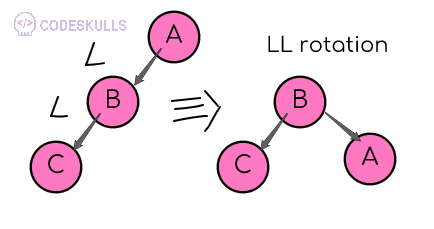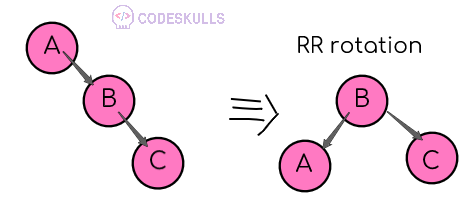# AVL Tree Rotations

In AVL tree, after performing the operations like insertions and deletions we need to check the balance factor of each node in a tree. if every node satisfies the binary factor condition then the tree is AVL and operations can be performed on that tree, if the tree doesn’t satisfy the binary factor condition then, the tree should be balanced using tree rotations

when the tree becomes imbalanced due to any operations, then tree rotations are used to make it balanced

Rotation is the process of moving the nodes either to the left or right to make the tree balanced

AVL Tree Rotations are Four rotations classified into two types

1. Single rotation
1. LL rotation
2. RR rotation
2. Double Rotation
1. LR rotation
2. RL rotation

## LL Rotation (Single left Rotation)#In LL rotation every node move one position to the left in a clockwise direction

## RR Rotation (Single right Rotation)#In RR rotation every node move one position to the Right from the current position in an Anti-clockwise direction

## LR Rotation (RR+LL Rotation)#

The LR rotation is the sequence of single right rotation and single left rotation, In this LR rotation at every node moves one position left and one position to right from the current position## RL Rotation (LL+RR Rotation)#

The RL rotation is the sequence of single left rotation and single right rotation, In this RL rotation at every node moves one position right and one position to left from the current positionLast updated on by vishal devxo